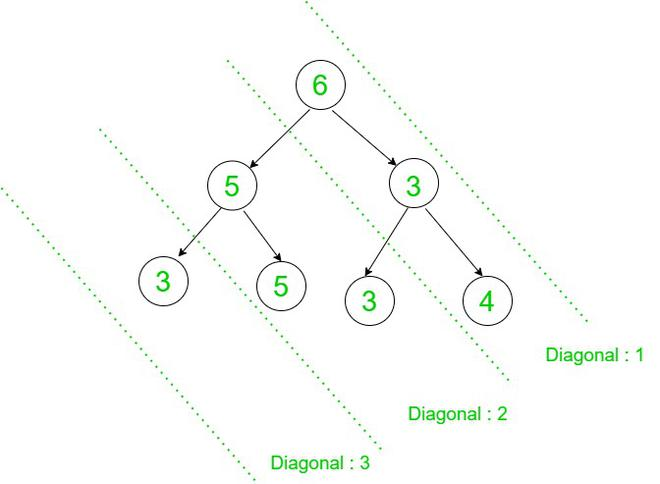# Compress a Binary Tree into an integer diagonally

• Last Updated : 18 Jun, 2021

Given a Binary Tree consisting of N nodes, the task is to first compress the tree diagonally to get a list of integers and then, again compress the list to get a single integer using the following operations:

• When a tree is compressed diagonally, its value in binary representation gets compressed.
• Consider each bit position of each node value present in a diagonal. If a position has S set bits and NS non-set bits, then set the bit for that position only if S is greater than NS. Otherwise, unset the bit for that position.
• Compress each diagonal to convert the tree into a list. Then, compress each array element into a single integer by using the same process.

Example: If 7, 6, 3 and 4 gets compressed, then their binary representations, i.e. (111)2, (110)2, (011)2 and (100)2 gets compressed. For the 0th position, S ≤ NS and for the 1st and 2nd positions, S > NS
Therefore, the number becomes (110)2 = 6.

Examples:

Input:               6
/      \
5          3
/     \      /    \
3        5   3       4
Output: 3
Explanation:Diagonal 1: Compress( 6, 3, 4 ) = 6
Diagonal 2: Compress( 5, 5, 3 ) = 5
Diagonal 3: Compress( 3 ) = 3
Finally, compress the list (6, 5, 3) to get 7.

Input:               10
/      \
5           2
/     \
6         8
Output: 2

Approach: The idea is to use a Hashmap to store all the nodes which belong to a particular diagonal of the tree. Follow the steps below to solve the problem:

• For the diagonal traversal of the tree, keep track of the horizontal distance from the root node for each node.
• Use a Hashmap to store the elements belonging to the same diagonal.
• After the traversal, count the number of set bits for each position for each diagonal of the tree and set the bit for the positions where the number of set bits exceeds the number of unset bits.
• Store the compressed value of each diagonal in an array.
• After obtaining the array, apply the same steps for compression to obtain the required integer.

Below is the implementation of the above approach:

## C++

 `#include ` `using` `namespace` `std;` `struct` `TreeNode{``  ``int` `val;``  ``TreeNode *left,*right;` `    ``TreeNode(``int` `v){``        ``val = v;``        ``left = NULL;``        ``right = NULL;``    ``}``};` `// Function to compress the elements``// in an array into an integer``int` `findCompressValue(vector<``int``> arr){``    ``int` `ans = 0;``    ``int` `getBit = 1;` `    ``// Check for each bit position``    ``for` `(``int` `i = 0; i < 32; i++){``        ``int` `S = 0;``        ``int` `NS = 0;` `        ``for` `(``int` `j:arr){` `            ``// Update the count of``            ``// set and non-set bits``            ``if` `(getBit & j)``                ``S += 1;``            ``else``                ``NS += 1;``          ``}` `        ``// If number of set bits exceeds``        ``// the number of non-set bits,``        ``// then add set bits value to ans``        ``if` `(S > NS)``            ``ans += ``pow``(2,i);` `        ``getBit <<= 1;``      ``}``    ``return` `ans;``}` `// Perform Inorder Traversal``// on the Binary Tree``void` `diagonalOrder(TreeNode *root,``int` `d,map<``int``,vector<``int``> > &mp){``    ``if` `(!root)``        ``return``;` `    ``// Store all nodes of the same``    ``// line together as a vector``    ``mp[d].push_back(root->val);` `    ``// Increase the vertical``    ``// distance of left child``    ``diagonalOrder(root->left, d + 1, mp);` `    ``// Vertical distance remains``    ``// same for right child``    ``diagonalOrder(root->right, d, mp);``}` `// Function to compress a given``// Binary Tree into an integer``int` `findInteger(TreeNode *root){` `    ``// Declare a map``    ``map<``int``,vector<``int``> > mp;` `    ``diagonalOrder(root, 0, mp);` `    ``//Store all the compressed values of``    ``//diagonal elements in an array``    ``vector<``int``> arr;` `    ``for` `(``auto` `i:mp)``        ``arr.push_back(findCompressValue(i.second));` `    ``// Compress the array into an integer``    ``return` `findCompressValue(arr);``}` `// Driver Code``// Given Input``int` `main()``{``  ``TreeNode *root = ``new` `TreeNode(6);``  ``root->left = ``new` `TreeNode(5);``  ``root->right = ``new` `TreeNode(3);``  ``root->left->left = ``new` `TreeNode(3);``  ``root->left->right = ``new` `TreeNode(5);``  ``root->right->left = ``new` `TreeNode(3);``  ``root->right->right = ``new` `TreeNode(4);` `  ``// Function Call``  ``cout << findInteger(root);` `  ``return` `0;``}` `// This code is contributed by mohit kumar 29.`

## Python3

 `# Python program for the above approach` `class` `TreeNode:` `    ``def` `__init__(``self``, val ``=``'',``                 ``left ``=` `None``,``                 ``right ``=` `None``):``        ``self``.val ``=` `val``        ``self``.left ``=` `left``        ``self``.right ``=` `right` `# Function to compress the elements``# in an array into an integer``def` `findCompressValue(arr):``    ``ans ``=` `0``    ``getBit ``=` `1` `    ``# Check for each bit position``    ``for` `i ``in` `range``(``32``):``        ``S ``=` `0``        ``NS ``=` `0` `        ``for` `j ``in` `arr:` `            ``# Update the count of``            ``# set and non-set bits``            ``if` `getBit & j:``                ``S ``+``=` `1``            ``else``:``                ``NS ``+``=` `1` `        ``# If number of set bits exceeds``        ``# the number of non-set bits,``        ``# then add set bits value to ans``        ``if` `S > NS:``            ``ans ``+``=` `2``*``*``i` `        ``getBit <<``=` `1` `    ``return` `ans` `# Function to compress a given``# Binary Tree into an integer``def` `findInteger(root):` `    ``# Declare a map``    ``mp ``=` `{}` `    ``# Perform Inorder Traversal``    ``# on the Binary Tree``    ``def` `diagonalOrder(root, d, mp):``        ``if` `not` `root:``            ``return` `        ``# Store all nodes of the same``        ``# line together as a vector``        ``try``:``            ``mp[d].append(root.val)` `        ``except` `KeyError:``            ``mp[d] ``=` `[root.val]` `        ``# Increase the vertical``        ``# distance of left child``        ``diagonalOrder(root.left, d ``+` `1``, mp)` `        ``# Vertical distance remains``        ``# same for right child``        ``diagonalOrder(root.right, d, mp)` `    ``diagonalOrder(root, ``0``, mp)` `    ``# Store all the compressed values of``    ``# diagonal elements in an array``    ``arr ``=` `[]``    ``for` `i ``in` `mp:``        ``arr.append(findCompressValue(mp[i]))` `    ``# Compress the array into an integer``    ``return` `findCompressValue(arr)`  `# Driver Code``# Given Input``root ``=` `TreeNode(``6``)``root.left ``=` `TreeNode(``5``)``root.right ``=` `TreeNode(``3``)``root.left.left ``=` `TreeNode(``3``)``root.left.right ``=` `TreeNode(``5``)``root.right.left ``=` `TreeNode(``3``)``root.right.right ``=` `TreeNode(``4``)` `# Function Call``print``(findInteger(root))`

Output:

`7`

Time Complexity: O(N)
Auxiliary Space: O(N)

My Personal Notes arrow_drop_up